# Sample Data: Fisher's Irises

Fisher's iris data

The data set consists of 50 samples from each of three species of iris flowers (setosa, versicolor and virginica). Four features were measured from each flower, the length and the width of the sepal and petal. The test and training sets were constructed by stratified random sampling, using 30% of the data for the test set and the rest for the training set.

## Examples

### Basic Examples

Retrieve the resource:

 In:=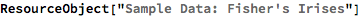Out=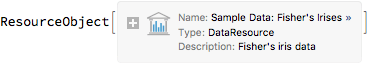View the data:

 In:=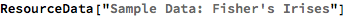Out=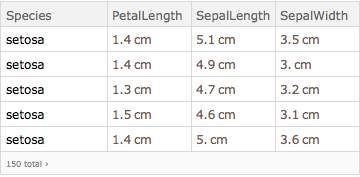### Visualization

Compare mean petal lengths for setosa, versicolor, and virginica:

 In:=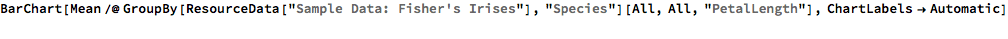Out=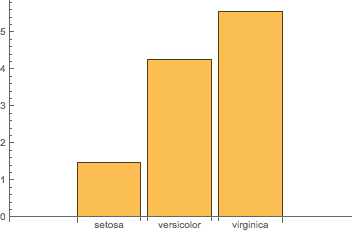### Analysis

Train a classifier:

 In:=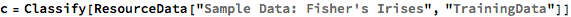Out=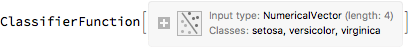Obtain general information about the classifier:

 In:=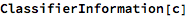Out=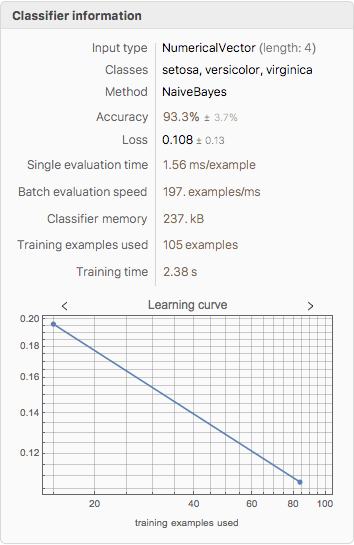Generate a ClassifierMeasurementsObject of the classifier with the test set:

 In:=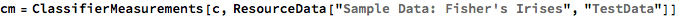Out=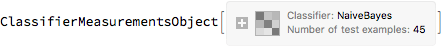Visualize the accuracy of the classifier:

 In:=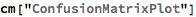Out=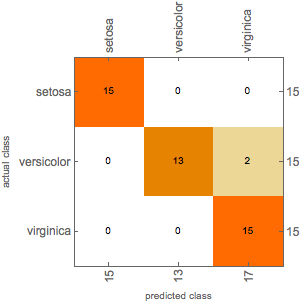Wolfram Research, "Sample Data: Fisher's Irises" from the Wolfram Data Repository (2018) https://doi.org/10.24097/wolfram.89373.data